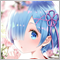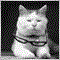# how to make my EA work with cryptocurrency?140

Evening people. Second time posting and the feedback is incredible really pushing my learning along.

so my final issue with this EA is i backtest it yet it places no trades at all. Sometimes ill see it says "limit" trade and even then it wont be filled. Below is my code and any corrections and advic will be much appreciated.

```//+------------------------------------------------------------------+

//|                                                 MACMARS.mq4 |

//|                             |

//+------------------------------------------------------------------+

#property version   "1.00"

#property strict

#property show_inputs

#include  <customfunctions01.mqh>

int rsiPeriod = 7;

input int rsiLowerLevel = 25;

input int rsiUpperLevel = 85;

input int stoplosspips = 200;

input int takeprofitpips = 1000;

input int SlowMA = 200;

input int FastMA = 50;

input int StoUpperlevel = 85;

input int StoLowerLevel = 25;

input int ticket = 0;

input    string      TRAILING       = "========= TRAILING =========";

input    bool        useTS          = true;    // Use Trailing

input    double      tsstop         = 200;       // Trailing Stop

input    double      tsstart        = 200;       // Trailing Start

input    double      tsstep         = 200;        // Trailing Step

int openOrderID;

int magic = magicNB;

//+------------------------------------------------------------------+

//| Expert initialization function                                   |

//+------------------------------------------------------------------+

int OnInit()

{

return(INIT_SUCCEEDED);

}

//+------------------------------------------------------------------+

//| Expert deinitialization function                                 |

//+------------------------------------------------------------------+

void OnDeinit(const int reason)

{

}

//+------------------------------------------------------------------+

//| Expert tick function                                             |

//+------------------------------------------------------------------+

void OnTick()

{

InitiateTrailing(useTS);

double FastMA = iMA(NULL,0,50,0,MODE_SMA,PRICE_CLOSE,0);

double SlowMA= iMA(NULL,0,200,0,MODE_SMA,PRICE_CLOSE,1);

double rsiValue = iRSI(NULL,0,rsiPeriod,PRICE_CLOSE,0);

double currentRSI = iRSI(NULL,0,7,0,0);

double previousRSI = iRSI(NULL,0,7,0,5);

double Stovalue = iStochastic(NULL, 0, 14 , 3, 3, MODE_SMA, MODE_MAIN, 0, 0);

double currentStovalue = iStochastic(NULL, 0, 14 , 3, 3, MODE_SMA, MODE_MAIN, 0, 0);

double previousStovalue = iStochastic (NULL, 0, 14 , 3, 3, MODE_SMA, MODE_MAIN, 0, 1);

double MACD = iMACD(NULL,0,12,26,9,PRICE_CLOSE,MODE_MAIN,0);

if(!checkifopenordersbymagicnb(magicNB))//if no open orders try to enter new position

// if (isnewcandle())

{

if (rsiValue<15 &&  FastMA>SlowMA && Bid<SlowMA) //buying

{

Print("MA's show a bullish market and rsiValue is lower than " + rsiLowerLevel+ " , Sending buy order");

double stopLossPrice = Bid - stoplosspips * getpipvalue();

double takeProfitPrice = Bid + takeprofitpips * getpipvalue();

Print("Entry Price = " + Bid);

Print("Stop Loss Price = " + stopLossPrice);

Print("Take Profit Price = " + takeProfitPrice);

if(openOrderID < 0) Alert("order rejected. Order error: " + GetLastError());

}

else if(rsiValue > 85  &&  SlowMA>FastMA  && Ask>SlowMA )//shorting

{

Print("MA's show a bearish market and rsiValue is above  " + rsiUpperLevel+ " , Sending short order");

double stopLossPrice = Ask + stoplosspips * getpipvalue();

double takeProfitPrice = Ask - takeprofitpips * getpipvalue();

Print("Entry Price = " + Ask);

Print("Stop Loss Price = " + stopLossPrice);

Print("Take Profit Price = " + takeProfitPrice);

if(openOrderID < 0) Alert("order rejected. Order error: " + GetLastError());

}

}

}

double PipsToPrice(double pips)

{

double PointValue;

if (Digits == 5 || Digits == 3) PointValue = 10.0 * Point;

else PointValue = Point;

if (Symbol() == "GOLD" || Symbol() == "SILVER" || Digits==2)

{

return pips/10;

PointValue = Point*10;// / 100.0;

}

if (Symbol() == "GOLDEURO"|| Symbol() == "SILVEREURO")

{

PointValue = Point;

}

return pips*PointValue;

double P=1;

if(Digits==5 || Digits==3)P=10;else P=1;

return pips*(Point*P);

}

//+------------------------------------------------------------------+

//| Trailing Functions                                               |

//+------------------------------------------------------------------+

void InitiateTrailing(bool allowTS)

{

if(allowTS)

{

TrailingPositionSell(tsstop,tsstart,tsstep);

}

}

//+------------------------------------------------------------------+

{

for(int i=0;i<OrdersTotal();i++)

{

if (OrderSelect(i, SELECT_BY_POS)  &&  (OrderMagicNumber()==magic)   &&  OrderSymbol()== Symbol() )

{

if(NormalizeDouble((Bid-OrderOpenPrice()),Digits)>PipsToPrice(activatePoint))

{

{

if(OrderStopLoss()==0)

{

ModifyStopLoss(Bid-trailingStop*Point*200);

}

else if(OrderStopLoss()<Bid-trailingStop*Point*200)

{

//ModifyStopLoss(Bid-trailingStop*Point*10);

ModifyStopLoss(OrderStopLoss()+(step)*Point*200);

}

}

}

}

}

}

//+------------------------------------------------------------------+

void TrailingPositionSell(double trailingStop,double activatePoint,double step)

{

for(int i=0;i<OrdersTotal();i++)

{

if (OrderSelect(i, SELECT_BY_POS)  &&  (OrderMagicNumber()== magic)   &&  OrderSymbol()== Symbol() )

{

{

if(OrderType()==OP_SELL)

{

if(OrderStopLoss()==0)

{

}

{

ModifyStopLoss(OrderStopLoss()-step*Point*200);

}

}

}

}

}

}

//+------------------------------------------------------------------+

void ModifyStopLoss(double sl)

{

bool fm;

fm = OrderModify(OrderTicket(),OrderOpenPrice(),sl,OrderTakeProfit(),0);

}

//+------------------------------------------------------------------+  ```1304

try to use 1lot instead of 0.01? some brokers have minimal lotsize for crypto535

0.01 is very common minimum lotsize, and also lot step, however, i recommend that you check the symbol in MarketWatch for the lot step and the minimum and maximum lot allowed. From there you can use lotstep to equal a trade size that you can be confident that the broker will allow.

EDIT the reason that your Limit order is not being filled is because you are using the current bid and ask price. LIMIT and STOP orders can not be the current Bid and Ask prices.

if you want to use Bid and Ask prices, then use market orders OP_BUY or OP_SELL instead. You can search the site for difference between market orders and the other 2 types.140

0.01 is very common minimum lotsize, and also lot step, however, i recommend that you check the symbol in MarketWatch for the lot step and the minimum and maximum lot allowed. From there you can use lotstep to equal a trade size that you can be confident that the broker will allow.

EDIT the reason that your Limit order is not being filled is because you are using the current bid and ask price. LIMIT and STOP orders can not be the current Bid and Ask prices.

if you want to use Bid and Ask prices, then use market orders OP_BUY or OP_SELL instead. You can search the site for difference between market orders and the other 2 types.

hey! thanks for taking time out of your day to advise... Really appreciate it. i will be doing this now, many thanks140

Pak Hong Poon #:
try to use 1lot instead of 0.01? some brokers have minimal lotsize for crypto